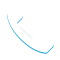### IBM SPSS Modeler for IBM Cloud Pak for Data (V3.0.x) eLearning – 6X334G WBT

Details

Course Code: 6X334G

Brand: IBM Cloud Pak for Data

Category: Analytics

Skill Level: Intermediate

Duration: 6.00H

Modality: WBT

Audience

• Clients who are new to IBM SPSS Modeler for IBM Cloud Pak for Data or want to find out more about using it

Prerequisites

• Knowledge of your business requirements

Short Summary

Students will learn how to import, explore, and prepare data, and introduces the student to machine learning models with SPSS Modeler for Cloud Pak for Data.

Overview

IBM SPSS Modeler is a premium service for IBM Cloud Pak for Data V3.0.x This course reviews the basics of how to import, explore, and prepare data, and introduces the student to machine learning models with SPSS Modeler for Cloud Pak for Data.

Topic

Introduction to SPSS Modeler for IBM Cloud Pak for Data
• Introduction to data science
• Describe the CRISP-DM methodology
• Introduction to SPSS Modeler
• Build models and apply them to new data

Import and explore
the data

• Describe key terms in working with data
• Import and export data
• Audit the data
• Define missing values

Integrate data
• Identify the unit of analysis
• Remove duplicate records and aggregate data
• Append and merge datasets
• Append and merge datasets with incomplete data

Transform fields
• Use the Control Language for Expression Manipulation
• Derive fields
• Use functions
• Reclassify fields

Identify relationships
• Overview of the nodes to use
• Explore the relationship between two categorical fields
• Explore the relationship between a categorical field and a continuous field
• Explore the relationship between two continuous fields

Introduction to modeling
• Identify three types of machine learning models
• Identify three types of supervised models
• Identify unsupervised models
• Deploy machine learning models

Objectives

• Gain introductory knowledge of SPSS Modeler for IBM Cloud Pak for Data
• Learn to import, integrate and explore the data
• Transform fields and identify relationships
• Get an introduction to machine learning models

Tags :Call Now +27 72 266 2599